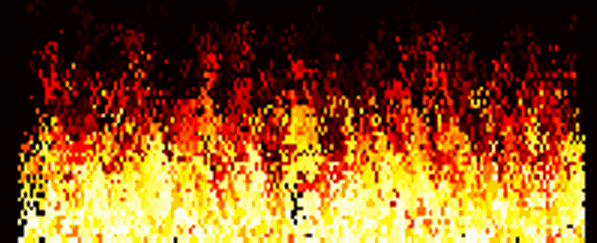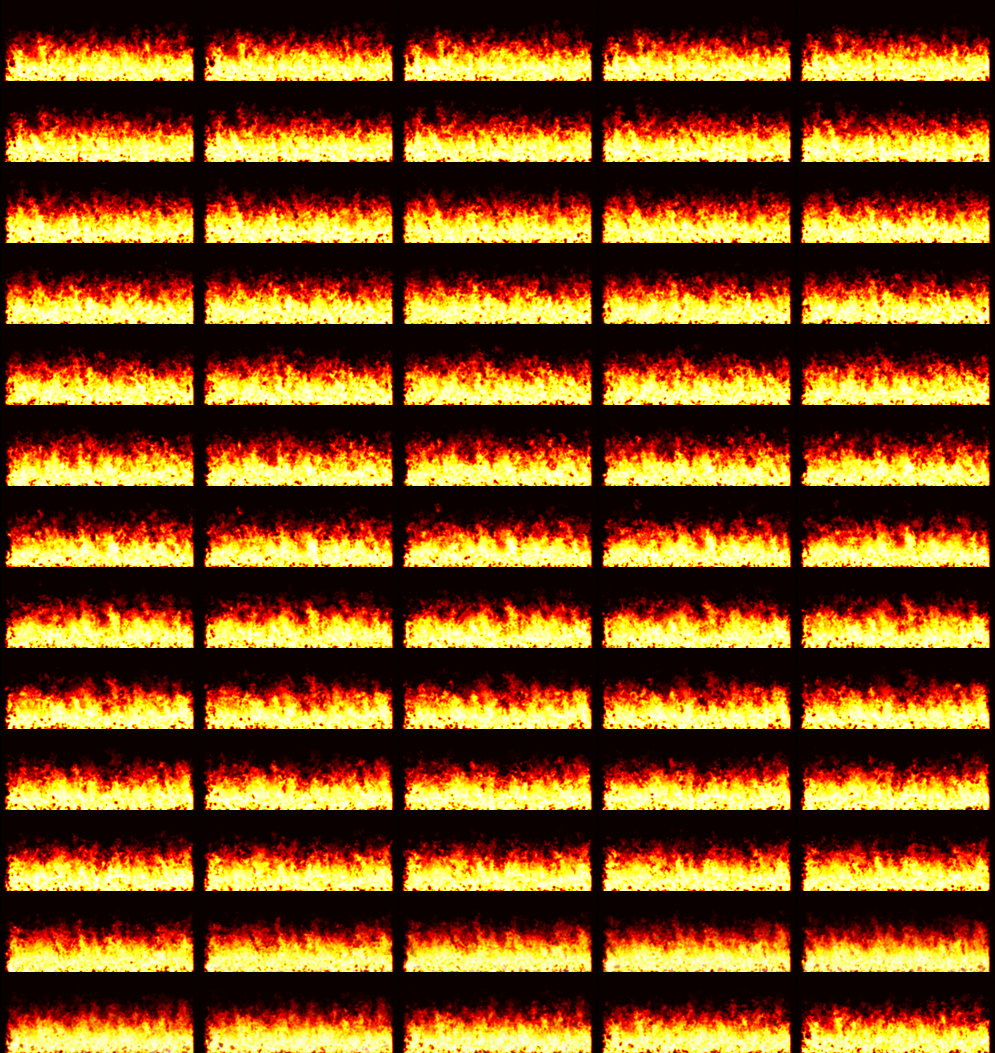# Realistic Fire Simulation using Cellular Automata

In this post I will explain how you can create an interesting fire effect using simple “game of life”-like rules and some post processing and why it is not a good idea to do this in real time.

I originally created this fire simulation to add an improved fire simulation in my project in XNA. The animation went from being created in “real time” on the GPU, to be created as a offline solution.

Here is the effect:The idea behind the fire is to start the matrix M with all zeros. For each frame in the animation, calculate the following:

```M(end-k:end, : ) = 0.5*M(end-k:end, : ) + 0.5*(rand(k+1, n) < 0.2);
```

This is a random k pixel wide seed that is “feeding” the fire from below, much like adding gasoline to the fire. The main loop (scale=6). The rest of the variables should be experimented on to get right, because they depend on the size of the animation.

```for i = scale+1:m-scale
for j = scale+1:n-scale
num = sum( sum( M(i-scale:i+scale, j-scale:j+scale) > 0.4) );
%calculate heat for each pixel

%Horizontal coupling
if num <= 7*scale
offset_j = (rand - 1);
offset_i = (rand - 1);

M_tmp(i, j) = 0.93*M(i+round(offset_i), j+round(offset_j));
end

%How long the flames stay alive and how they communicate when they die
if num <= 2*scale
offset = (2*rand-1); %Additional heat haze effect
offset_exp = 1.3;
M_tmp(i, j) = M(i + abs(round(3*offset)), j+round(offset))^offset_exp;
%added horizontal jitter
end
end
end
```

These two rules makes the fire dissipate in a convincing way, occasionally creating smaller flicking flames. The values of the matrix are mapped to red/yellow colors.

To simulate the upwards motion of a flame caused by heat, we simply move every element in the matrix upwards:

M_tmp(1:m-1, : ) = M_tmp(2:m, : );

Some adjustments are made to the color mapping:

Mp=M_tmp.^0.05; %map into harsher colors

Unfortunately, we are not finished yet, the raw matrix does not produce a really convincing fire simulation as you can see:Anyway, more on that later. Worth mentioning is that the first idea was to implement this code in HLSL in XNA. I created a render target to do the updating of the texture and sending it back to the GPU. This is wasteful because this is basically synchronizing the CPU and GPU, stalling the pipeline.

So, my solution was to create the fire simulation and store the frames in a sprite, send to the GPU once and cycle through the animation:Because the GPU can only hold so much data, the animation only lasts about 50 frames or so. The solution is to make use of the data I have and make the animation seamless.

One of the attempts was to wrap the frames around and add random noise in increasing order until the last frame was the same as the first frame. This didn’t look very good because of the crispness of the flames. I settled with applying a simple linear fade between the first ten and last ten frames. The idea is to start at frame 10 and move forward, then interpolate the first 10 frames with the last 10 frames of the animation. I called the function linearburn, it simply takes base image a, target image b and strength of the burn r and applies the filter, making the animation seamless.

```function linearburn(a, b, r)

for k = 1:3
a(:, :, k) = r.*a(:, :, k) + (1-r).*b(:, :, k);
end
```

I believe, to get a really convincing fire animation, the fading should also animate. The final result of the experiments can be seen below:I am really pleased with the results. The main idea was to create a fire animation using a simple idea and it worked. If the animation is in real time it is worth mentioning that the offset values can simulate wind. It would be nice also to increase the resolution of the animation somehow. I also found that it is possible to create other interesting animations, but that is for another time.

### 2 Comments:

1.You should add a dynamic Javascript/WebGL version of the fire CA for your visitors to play with.

•Yes, sounds good.